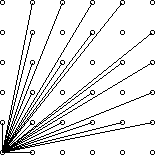# coreBugZJ

## POJ 3090. Visible Lattice Points

Visible Lattice Points
 Time Limit: 1000MS Memory Limit: 65536K Total Submissions: 4099 Accepted: 2288

Description

A lattice point (x, y) in the first quadrant (x and y are integers greater than or equal to 0), other than the origin, is visible from the origin if the line from (0, 0) to (x, y) does not pass through any other lattice point. For example, the point (4, 2) is not visible since the line from the origin passes through (2, 1). The figure below shows the points (x, y) with 0 ≤ x, y ≤ 5 with lines from the origin to the visible points.Write a program which, given a value for the size, N, computes the number of visible points (x, y) with 0 ≤ x, yN.

Input

The first line of input contains a single integer C (1 ≤ C ≤ 1000) which is the number of datasets that follow.

Each dataset consists of a single line of input containing a single integer N (1 ≤ N ≤ 1000), which is the size.

Output

For each dataset, there is to be one line of output consisting of: the dataset number starting at 1, a single space, the size, a single space and the number of visible points for that size.

Sample Input

```4
2
4
5
231```

Sample Output

```1 2 5
2 4 13
3 5 21
4 231 32549```

Source

Greater New York 2006

1/*
2POJ 3090 Visible Lattice Points
34Farey 数列，欧拉函数。
5*/

678#include <stdio.h>
9#include <string.h>
1011#define  N  1003
1213int prime[ N ], nprime;
14int fun[ N ], ans[ N ];
1516void init_prime() {
17int i, j;
18memset( prime, 0sizeof(prime) );
19nprime = 0;
20for ( i = 2; i < N; ++i ) {
21if ( 0 == prime[ i ] ) {
22prime[ nprime++ ] = i;
23for ( j = i+i; j < N; j+=i ) {
24prime[ j ] = 1;
25}

26}

27}

28}

2930void init_fun() {
31int  i, j;
32int t;
33for ( i = 1; i < N; ++i ) {
34t = i;
35for ( j = 0; (j < nprime)&&(prime[j]<=i); ++j ) {
36if ( i % prime[j] == 0 ) {
37t = t * (prime[ j ] - 1/ prime[ j ];
38}

39}

40fun[ i ] = t;
41}

42fun[ 1 ] = 0;
43}

4445void init_ans() {
46int i;
47ans[ 1 ] = 0;
48for ( i = 2; i < N; ++i ) {
49ans[ i ] = ans[ i - 1 ] + fun[ i ];
50}

51for ( i = 1; i < N; ++i ) {
52ans[ i ] = ans[ i ] * 2 + 3;
53}

54}

5556int main() {
57int n, c, i;
58init_prime();
59init_fun();
60init_ans();
6162scanf( "%d"&c );
63for ( i = 1; i <= c; ++i ) {
64scanf( "%d"&n );
65printf( "%d %d %d\n", i, n, ans[n] );
66}

67return 0;
68}

69posted on 2012-02-29 21:16 coreBugZJ 阅读(276) 评论(0)  编辑 收藏 引用 所属分类: ACMAlgorithmMathematics